# Tutorial: Bayesian Structural Time Series Model

This tutorial demonstrates modeling and running inference on various Bayesian Structural Time Series (STS) models. We demonstrate some great features of Bean Machine such as: modeling serially correlated variables, the NUTS sampler applied to a global pool of variables, and providing custom distributions for inference.

import sysimport osif 'google.colab' in sys.modules and 'beanmachine' not in sys.modules:    !pip install beanmachinesmoke_test = ('SANDCASTLE_NEXUS' in os.environ or 'CI' in os.environ)

# Problem

Bayesian STS is a general class of additive models for Time Series series data; $y_1, y_2, ..., y_n$ that have an associated State Space denoted by; $\alpha_1, \alpha_2, ..., \alpha_n$ which provides a probabilistic model for the serial correlation observed in the time series. For this tutorial, we will consider the case when $\alpha_i$ takes values in a continous state space (For a discussion of the discrete state space case, refer to this tutorial on Hidden Markov Models).

Bayesian STS models typically contain, but are not limited to, some additive mixture of Error ($\epsilon$), Trend ($\mu$), and Seasonality ($\gamma$) components: $$y_t = \mu_t + \gamma_t + \epsilon_t$$ Where each component is controlled either by some recursive relation of the state space, $\alpha_i$ or external covariates (i.e. Regression) such as day-of-week effects, business logic, or categories of the data. When STS just contains just the Error, Trend, and Seasonality components, it is sometimes referred to as the ETS model. Possibly the simpliest version of STS is called the local level model which is where we will start our discussion after some prerequisites.

# Prerequisites

Let's code this in Bean Machine! Import the Bean Machine library, some fundamental PyTorch classes, and optionally typing for our code.

import loggingfrom typing import Listimport beanmachine.ppl as bmimport matplotlib.pyplot as pltimport numpy as npimport pandas as pdimport torchimport torch.distributions as distfrom beanmachine.ppl.model import RVIdentifierlogging.getLogger("beanmachine").setLevel(50)bm.seed(111)

# Local Level Model

In this simple local level version of an STS, $y_t$ is the sum of our state variable $\alpha_t$ plus Gaussian Noise, $\epsilon_t$. All of the time dependency is modeled in the evolution of $\alpha_{t+1}$, which is equal to its previous time value $\alpha_t$ plus Gaussian Noise, $\eta_t$ that is independent of all $\epsilon_i$'s. This is sometimes referred to a random walk because at each step, noise is injected to the previous state to arrive at a new state. To summarize, the random variables in play at this point are:

• $\epsilon_t \stackrel{iid}{\sim} \text{Normal}(0, \sigma_\epsilon^2)$
• $\eta_t \stackrel{iid}{\sim} \text{Normal}(0, \sigma_\eta^2)$
• $\eta_t \perp \epsilon_i, \forall i \in \{1,..., n\}$

Most STS formulations are expressed in the state space representation, which is a coupled set of equations known as the measurement and state equations. Using our notation from before, the local level state space representation is:

NameFormula
Measurement Equation$y_t = \alpha_t + \epsilon_t$
State Equation$\alpha_{t+1} = \alpha_t + \eta_t$

This can be represented more compactly as:

• $\alpha_{t+1} | \alpha_t \sim \text{Normal}(\alpha_{t}, \sigma_\eta^2)$
• $y_t | \alpha_t \sim \text{Normal}(\alpha_t, \sigma_e^2)$

To make our STS "Bayesian", we will assign priors to $\sigma_\epsilon$, $\sigma_\eta$, and our initial state $\alpha_1$:

• $\sigma_\epsilon \sim \text{Gamma}(\epsilon_{\text{shape}}, \epsilon_{\text{rate}})$
• $\sigma_\eta \sim \text{Gamma}(\eta_{\text{shape}}, \eta_{\text{rate}})$
• $\alpha_1 \sim \text{Normal}(a_1, p_1^2)$

We can implement this model in Bean Machine by defining random variable objects with the @bm.random_variable decorator. These functions behave differently than ordinary Python functions.

Semantics for @bm.random_variable functions:

• They must return PyTorch Distribution objects.
• Though they return distributions, callees actually receive samples from the distribution. The machinery for obtaining samples from distributions is handled internally by Bean Machine.
• Inference runs the model through many iterations. During a particular inference iteration, a distinct random variable will correspond to exactly one sampled value: calls to the same random variable function with the same arguments will receive the same sampled value within one inference iteration. This makes it easy for multiple components of your model to refer to the same logical random variable.
• Consequently, to define distinct random variables that correspond to different sampled values during a particular inference iteration, an effective practice is to add a dummy "indexing" parameter to the function. Distinct random variables can be referred to with different values for this index.

Note also that, compared to the statistical notation above, our implementation uses 0-indexing instead of 1-indexing.

class LocalLevelModel:    def __init__(        self,        N: int,        epsilon_shape: float,        epsilon_rate: float,        eta_shape: float,        eta_rate: float,        a_1: float,        p_1: float,    ) -> None:        self.N = N        self.epsilon_shape = epsilon_shape        self.epsilon_rate = epsilon_rate        self.eta_shape = eta_shape        self.eta_rate = eta_rate        self.a_1 = a_1        self.p_1 = p_1    @bm.random_variable    def SigmaEpsilon(self) -> RVIdentifier:        return dist.Gamma(self.epsilon_shape, self.epsilon_rate)    @bm.random_variable    def SigmaEta(self) -> RVIdentifier:        return dist.Gamma(self.eta_shape, self.eta_rate)    @bm.random_variable    def Alpha(self, n: int) -> RVIdentifier:        if n == 0:            return dist.Normal(self.a_1, self.p_1)        else:            return dist.Normal(self.Alpha(n - 1), self.SigmaEta())    @bm.random_variable    def Y(self, n: int) -> RVIdentifier:        return dist.Normal(self.Alpha(n), self.SigmaEpsilon())

## Data​

iclaims is a dataset containing the weekly initial claims for US unemployment benefits against a few related google trend queries (unemploy, filling and job)from Jan 2010 - June 2018. This dataset was curated here and credit goes towards Uber's Orbit package.

def load_iclaims(end_date='2018-06-24', transform=True):    """Load iclaims dataset    Returns    -------        pd.DataFrame    Notes    -----    iclaims is a dataset containing the weekly initial claims for US unemployment benefits against a few related google    trend queries (unemploy, filling and job)from Jan 2010 - June 2018. This aims to mimick the dataset from the paper    Predicting the Present with Bayesian Structural Time Series by SCOTT and VARIAN (2014).    Number of claims are obtained from [Federal Reserve Bank of St. Louis] while google queries are obtained through    Google Trends API.    Note that dataset is transformed by natural log before fitting in order to be fitted as a multiplicative model.    https://fred.stlouisfed.org/series/ICNSA    https://trends.google.com/trends/?geo=US    https://finance.yahoo.com/    """    url = 'https://raw.githubusercontent.com/uber/orbit/master/examples/data/iclaims_example.csv'    df = pd.read_csv(url, parse_dates=['week'])    df = df[df['week'] <= end_date]    # standardize the regressors by mean; equivalent to subtracting mean after np.log    regressors = ['trend.unemploy', 'trend.filling', 'trend.job', 'sp500', 'vix']    # convert to float    for col in regressors:        df[col] = df[col].astype(float)    if transform:        # log transfer        df[['claims'] + regressors] = df[['claims'] + regressors].apply(np.log)        # de-mean        df[regressors] = df[regressors] - df[regressors].apply(np.mean)    return df
y_obs = torch.tensor(load_iclaims().claims.values)[:40]plt.plot(y_obs)plt.show()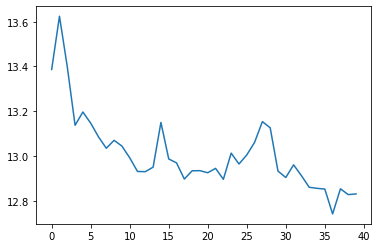## Inference​

Inference is the process of combining model with data to obtain insights, in the form of probability distributions over values of interest. Bean Machine offers a powerful and general inference framework to enable fitting a arbitrary models to data. First lets setup our priors and instantiate our model.

# Prior Specificationepsilon_shape = 0.5epsilon_rate = 1.0eta_shape = 0.5eta_rate = 1.0a_1 = y_obs.mean().item()p_1 = 1.0# Length of our time seriesN = len(y_obs)
# Instantiate the model and pass our length and priorsmodel = LocalLevelModel(    N=N,    epsilon_shape=epsilon_shape,     epsilon_rate=epsilon_rate,     eta_shape=eta_shape,    eta_rate=eta_rate,     a_1=a_1,    p_1=p_1 )

Running inference consists of a few arguments:

NameUsage
queriesA list of @bm.random_variable targets to fit posterior distributions for.
observationsThe Dict of observations we built up, above.
num_samplesNumber of samples to build up distributions for the values listed in queries.
num_chainsNumber of separate inference runs to use. Multiple chains can verify inference ran correctly.

The next step is to define the queries and observations. For this particular run, we're interested in infering $\alpha$, $\sigma_\epsilon$, and $\sigma_\eta$.

queries = [model.Alpha(n) for n in range(model.N)] + [model.SigmaEpsilon(), model.SigmaEta()]observations = {**{model.Y(n): y_obs[n] for n in range(model.N)}}

For this particular problem, we will use the GlobalNoUTurnSampler inference method. Bean Machine's GlobalNoUTurnSampler is the NUTS sampler you have probably used before with stan or pymc3. We have chosen to use the NUTS sampler here because it can be easily compared to other probabilistic tools.

num_samples = 400 if not smoke_test else 1num_adaptive_samples = 50all_samples = bm.GlobalNoUTurnSampler(max_tree_depth=10).infer(    queries,    observations,    num_samples,    num_chains=1,    num_adaptive_samples=num_adaptive_samples)samples = all_samples.get_chain(0)
Out:
Samples collected:   0%|          | 0/450 [00:00<?, ?it/s]
alpha_samples = torch.stack([samples[model.Alpha(n)] for n in range(model.N)], dim=1)

## Visualization​

We will look at the values of the samples collected for $\alpha$ and $\sigma_\epsilon$. We will take the mean of samples taken over the last 10% of the chain, and compare these to our data.

tail_len = num_samples // 10
ppc = np.percentile(  alpha_samples[-tail_len:].numpy(),  q=[5, 50, 95],  axis=0).Tplt.figure(figsize=(12,6))plt.plot(y_obs, label='Observations', color='green')plt.plot(ppc[:, 1], label=r'Median of $\alpha_t$', color='red')plt.plot(ppc[:, 0], 'b--', label=r'$5th$ Percentile')plt.plot(ppc[:, 2], 'b--', label=r'$95th$ Percentile')plt.title(r"Observations versus $\alpha_t$")plt.legend()plt.show()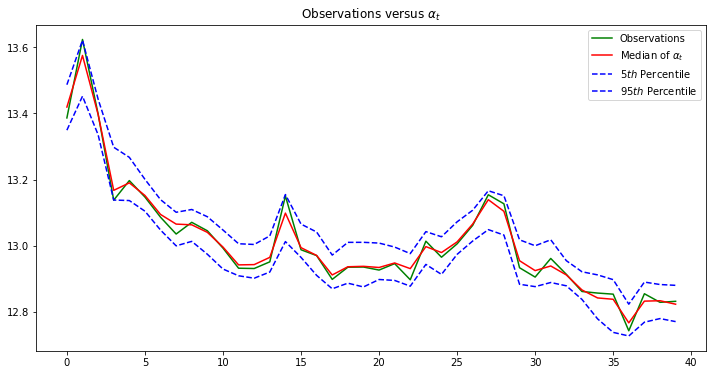It appears inference has recovered the latent states $\alpha$ very well, and is accurately representing $Y$ when sampled.

Now we investigate chain mixing by looking at the last half of the sampling chain. This type of plot is called a trace plot and is useful to visualize where the sampler is proposing steps.

plt.figure(figsize=(12,6))[plt.plot(alpha_samples[-num_samples//2:, i], label=f'Time index {i}') for i in [0, 10, 20, 30, 39]]plt.legend()plt.title(r"Trace Plots for $\alpha_t$")plt.show()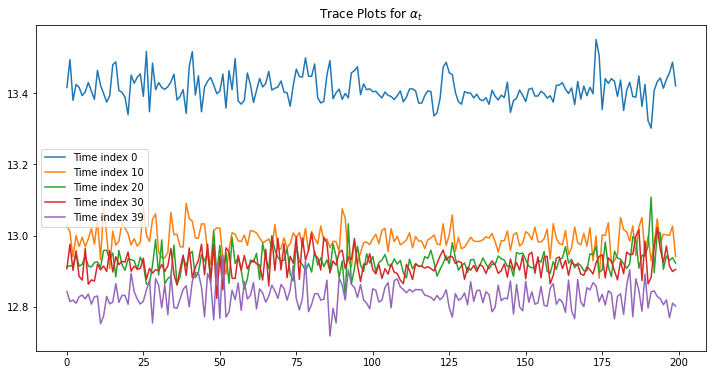We see a clear downward trend for successive time indices. This matches our intuition from the "Observations versus $\alpha_t$" plot as we see the original time series with a downward trend.

Finally we look at the uncertainty estimates as a function of time. These are the posterior samples for both $\sigma_\eta$ and $\sigma_\epsilon$.

plt.figure(figsize=(12,6))plt.plot(samples[model.SigmaEta()], label=r"State Uncertainty, $\sigma_\eta$")plt.plot(samples[model.SigmaEpsilon()], label=r"Measurement Uncertainty, $\sigma_\epsilon$")plt.title("Uncertainty")plt.legend()plt.show()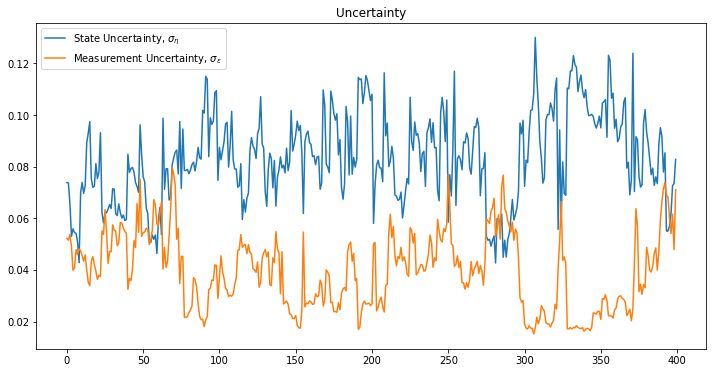We see the final noise samples are quite small, reflecting the fact that the original data did not contain alot of noise.

## Posterior Likelihood Checks​

One way to evaluate posterior samples is computing likelihoods of our posterior samples, and comparing these to the likelihood of the underlying data. Formally, we can compute the joint likelihood of posterior samples with the observations.

def log_likelihood(alpha_samples, sigma_epsilon, y_obs, N):    ll = 0    for n in range(N):        ll += dist.Normal(alpha_samples[n], sigma_epsilon).log_prob(y_obs[n])    return ll# Iterate over the batches of samples and compute log likelihoodlls = [    log_likelihood(alpha_samples, sigma_epsilon_sample, y_obs, N)    for alpha_samples, sigma_epsilon_sample in zip(alpha_samples, samples[model.SigmaEpsilon()])]
plt.figure(figsize=(12,6))plt.plot(lls, label="Sample", c="g")plt.title("Posterior Likelihood Check")plt.ylabel("Log likelihood")plt.xlabel("Iteration")plt.legend()plt.show()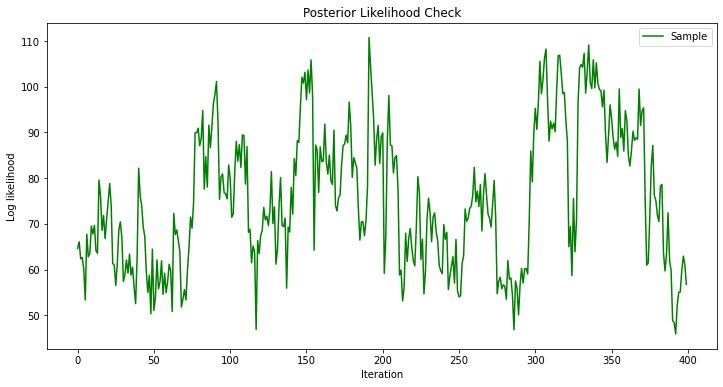From the above plot, inference appears to be fitting almost perfectly. The random variables given the observed data is exceptionally high.

# Vectorized Model

Now lets try a vectorized model by using a custom Distribution. We first extend pytorch's torch.distributions.Distribution into a vectorized version of a random walk. The key idea behind this implementation is that a Gaussian Random Walk can also be expressed as a chain of iid Gaussian random variables that have a cumsum operator applied to them (thus making the "walking" behavior). Now with this cumsum operator, we will be able to sample entire chains in one step!

from torch.distributions import Distribution, constraintsclass GaussianRandomWalk(Distribution):    support = constraints.real    def __init__(        self,        loc: torch.Tensor,        scale: torch.Tensor,        num_steps: int = 1,        validate_args=None,    ) -> None:        assert isinstance(num_steps, int) and num_steps > 0, "num_steps argument should be an positive integer."        self.loc = loc        self.scale = scale        self.num_steps = num_steps        batch_shape, event_shape = scale.size(), torch.Size([num_steps])        super(GaussianRandomWalk, self).__init__(batch_shape, event_shape, validate_args=validate_args)    def sample(self, sample_shape: List = None):        if not sample_shape:            sample_shape = []        assert isinstance(sample_shape, list), "sample_shape argument should be a list."        shape = torch.Size(sample_shape) + self.batch_shape + self.event_shape        loc = torch.zeros(shape)        loc[..., 0] = self.loc        walks = dist.Normal(loc=loc, scale=1).sample()        return torch.cumsum(walks, axis=-1) * self.scale.unsqueeze(dim=-1)    def log_prob(self, value):        init_prob = dist.Normal(self.loc, self.scale).log_prob(value[..., 0])        scale = self.scale.unsqueeze(dim=-1)        step_probs = dist.Normal(value[..., :-1], scale).log_prob(value[..., 1:])        return init_prob + torch.sum(step_probs, axis=-1)    @property    def mean(self):        return torch.zeros(self.batch_shape + self.event_shape) + self.loc    @property    def variance(self):        return torch.broadcast_to(            self.scale.unsqueeze(dim=-1) ** 2 * torch.arange(1, self.num_steps + 1),            self.batch_shape + self.event_shape,        )

In our implementation above, we will now use the sample() and log_prob() functions to seamlessly integrate into Bean Machine! Specifically, we replace the Alpha() function with our vectorized distribution rather than recursively calling the univariate function from before.

class VectorizedLocalLevelModel:    def __init__(        self, N: int, epsilon_shape: float, epsilon_rate: float, eta_shape: float, eta_rate: float, a_1: float    ) -> None:        self.N = N        self.epsilon_shape = epsilon_shape        self.epsilon_rate = epsilon_rate        self.eta_shape = eta_shape        self.eta_rate = eta_rate        self.a_1 = a_1    @bm.random_variable    def SigmaEpsilon(self) -> RVIdentifier:        return dist.Gamma(self.epsilon_shape, self.epsilon_rate)    @bm.random_variable    def SigmaEta(self) -> RVIdentifier:        return dist.Gamma(self.eta_shape, self.eta_rate)    @bm.random_variable    def Alpha(self) -> RVIdentifier:        return GaussianRandomWalk(loc=self.a_1, scale=self.SigmaEta(), num_steps=self.N)  # Vectorized!    @bm.random_variable    def Y(self) -> RVIdentifier:        return dist.Normal(self.Alpha(), self.SigmaEpsilon())  # Vectorized!

We keep the rest of the workflow to be very similar to the unvectorized model so that we can directly compare the results. We complete inference, visualize our samples, and then compute some diagnostics of the fitted values.

vec_model = VectorizedLocalLevelModel(    N=N,    epsilon_shape=epsilon_shape,    epsilon_rate=epsilon_rate,    eta_shape=eta_shape,    eta_rate=eta_rate,    a_1=a_1)queries = [vec_model.Alpha(), vec_model.SigmaEpsilon(), vec_model.SigmaEta()]observations = {**{vec_model.Y(): y_obs}}

One difference is that we now fit 4 chains (serially) in order to better investigate some diagnostic metrics. You can read more about this in the $\hat{R}$ and $ess$ cells located below.

num_samples = 400 if not smoke_test else 1vec_all_samples = bm.GlobalNoUTurnSampler(max_tree_depth=10).infer(    queries,    observations,    num_samples,    num_chains=4,    num_adaptive_samples=num_adaptive_samples,    verbose=False)
Out:
Samples collected:   0%|          | 0/450 [00:00<?, ?it/s]Samples collected:   0%|          | 0/450 [00:00<?, ?it/s]Samples collected:   0%|          | 0/450 [00:00<?, ?it/s]Samples collected:   0%|          | 0/450 [00:00<?, ?it/s]

The speedup is quite significant using a vectorized approach! One of the main benefits of using Bean Machine is being able to incorporate this type of fast code.

vec_samples = vec_all_samples.get_chain(0)vec_alpha_samples = vec_samples[vec_model.Alpha()]vec_alpha_samples.size()
Out:
torch.Size([400, 40])

## Visualization​

tail_len = num_samples // 10vec_ppc = np.percentile(  vec_alpha_samples[-tail_len:].numpy(),  q=[5, 50, 95],  axis=0).Tplt.figure(figsize=(12,6))plt.plot(y_obs, label='Observations', color='green')plt.plot(vec_ppc[:, 1], label=r'$\alpha_t$', color='red')plt.plot(vec_ppc[:, 0], 'b--', label=r'$5th$ Percentile')plt.plot(vec_ppc[:, 2], 'b--', label=r'$95th$ Percentile')plt.title(r"Observations versus $\alpha_t$")plt.legend()plt.show()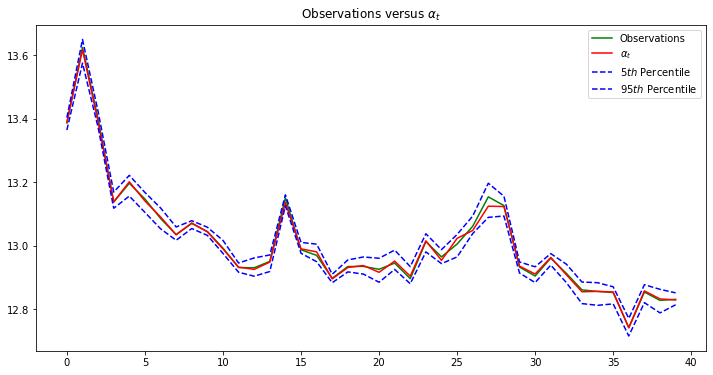plt.figure(figsize=(12,6))[plt.plot(vec_alpha_samples[-num_samples//2:, i], label=f'Time index {i}') for i in [0, 10, 20, 30, 38]]plt.legend()plt.title(r"Trace Plots for $\alpha_t$")plt.show()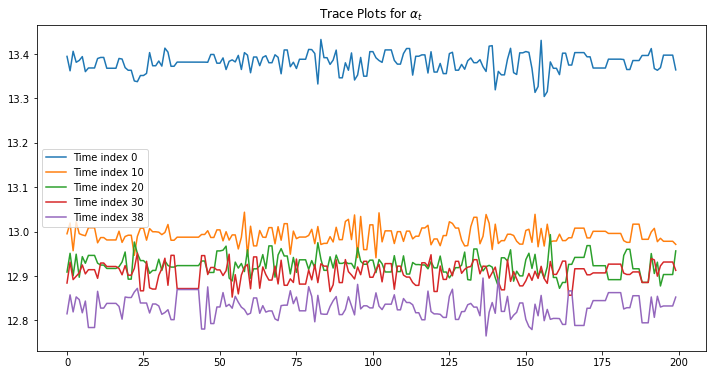plt.plot(vec_samples[vec_model.SigmaEta()], label=r"State Uncertainty, $\sigma_\eta$")plt.plot(vec_samples[vec_model.SigmaEpsilon()], label=r"Measurement Uncertainty, $\sigma_\epsilon$")plt.legend()plt.show()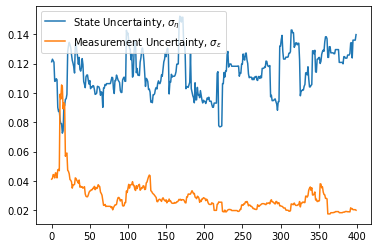## Diagnostics​

Two important statistics to take note of are the $\hat{R}$ (r_hat) and effective sample size (ess) values in the below dataframe, see Vehtari et al.

import arviz as azsummary_df = az.summary(vec_all_samples.to_xarray(), round_to=3)summary_df.T
SigmaEpsilon(<main.VectorizedLocalLevelModel object at 0x7fee105f6f50>,)Alpha(<main.VectorizedLocalLevelModel object at 0x7fee105f6f50>,)Alpha(<main.VectorizedLocalLevelModel object at 0x7fee105f6f50>,)Alpha(<main.VectorizedLocalLevelModel object at 0x7fee105f6f50>,)Alpha(<main.VectorizedLocalLevelModel object at 0x7fee105f6f50>,)Alpha(<main.VectorizedLocalLevelModel object at 0x7fee105f6f50>,)Alpha(<main.VectorizedLocalLevelModel object at 0x7fee105f6f50>,)Alpha(<main.VectorizedLocalLevelModel object at 0x7fee105f6f50>,)Alpha(<main.VectorizedLocalLevelModel object at 0x7fee105f6f50>,)Alpha(<main.VectorizedLocalLevelModel object at 0x7fee105f6f50>,)...Alpha(<main.VectorizedLocalLevelModel object at 0x7fee105f6f50>,)Alpha(<main.VectorizedLocalLevelModel object at 0x7fee105f6f50>,)Alpha(<main.VectorizedLocalLevelModel object at 0x7fee105f6f50>,)Alpha(<main.VectorizedLocalLevelModel object at 0x7fee105f6f50>,)Alpha(<main.VectorizedLocalLevelModel object at 0x7fee105f6f50>,)Alpha(<main.VectorizedLocalLevelModel object at 0x7fee105f6f50>,)Alpha(<main.VectorizedLocalLevelModel object at 0x7fee105f6f50>,)Alpha(<main.VectorizedLocalLevelModel object at 0x7fee105f6f50>,)Alpha(<main.VectorizedLocalLevelModel object at 0x7fee105f6f50>,)SigmaEta(<main.VectorizedLocalLevelModel object at 0x7fee105f6f50>,)
mean0.02913.37313.59213.39413.15713.19213.14713.08813.0413.067...12.95412.91112.86312.85612.84712.75512.84612.82912.8320.113
sd0.0130.0320.0440.030.0320.030.0280.0290.0270.029...0.0290.0280.0280.0290.0270.0310.0290.0290.0290.014
hdi_3%0.00913.31513.51713.34113.09613.13513.09413.03612.99113.014...12.90112.84912.80712.79912.79312.69712.78712.77712.7820.09
hdi_97%0.05213.42913.65813.45413.21413.24613.20113.14813.09513.123...13.00812.96212.91512.90812.89912.81212.89712.88412.8920.14
mcse_mean0.0020.0020.0050.0010.0020.0010.0010.0010.0010.001...0.0010.0010.0010.0010.0010.0010.0010.0010.0010.001
mcse_sd0.0020.0010.0030.0010.0020.0010.0010.00100...000.001000.0010.0010.00100.001
ess_bulk21.341496.575124.0381102.35248.9951768.7664.5391714.351763.92192.1...1735.312262.761488.572141.011634.28684.4781478.131645.622114.61222.529
ess_tail51.428278.26138.976335.601225.552315.877600.174835.802732.362511.996...463.851331.508595.205642.63562.712373.118625.599679.928815.075448.152
r_hat1.1791.021.0351.0261.0211.0261.0211.0241.0321.011...1.0231.0421.0241.0221.0221.0121.0431.0171.0271.018

### $\hat{R}$ Diagnostic​

$\hat{R}$ is a diagnostic tool that measures the between- and within-chain variances. It is a test that indicates a lack of convergence by comparing the variance between multiple chains to the variance within each chain. If the parameters are successfully exploring the full space for each chain, then $\hat{R}\approx 1$, since the between-chain and within-chain variance should be equal. $\hat{R}$ is calculated as

$\hat{R}=\frac{\hat{V}}{W}$

where $W$ is the within-chain variance and $\hat{V}$ is the posterior variance estimate for the pooled rank-traces. The take-away here is that $\hat{R}$ converges towards 1 when each of the Markov chains approaches perfect adaptation to the true posterior distribution. We do not recommend using inference results if $\hat{R}>1.01$. More information about $\hat{R}$ can be found in the Vehtari et al paper.

### Effective sample size $ess$ diagnostic​

MCMC samplers do not draw independent samples from the target distribution, which means that our samples are correlated. In an ideal situation all samples would be independent, but we do not have that luxury. We can, however, measure the number of effectively independent samples we draw, which is called the effective sample size. You can read more about how this value is calculated in the Vehtari et al paper, briefly it is a measure that combines information from the $\hat{R}$ value with the autocorrelation estimates within the chains. There are many ways to estimate effective samples sizes, however, we will be using the method defined in the Vehtari et al paper.

The rule of thumb for ess_bulk is for this value to be greater than 100 per chain on average. Since we ran four chains, we need ess_bulk to be greater than 400 for each parameter. The ess_tail is an estimate for effectively independent samples considering the more extreme values of the posterior. This is not the number of samples that landed in the tails of the posterior. It is a measure of the number of effectively independent samples if we sampled the tails of the posterior. The rule of thumb for this value is also to be greater than 100 per chain on average.

# Heteroscedastic Model

In the previous sections, one core assumption made about the data generation process is that $\epsilon_t \stackrel{iid}{\sim} \text{Normal}(0, \sigma_\epsilon^2)$, meaning that all of the noise in our measurement equation was sampled the same way, from the same distribution. In the Statistics literature, this is known as homoscedastic noise. Instead, we could postulate a second latent random variable, $\beta_t$ that would capture some of the serial correlation in the noise over time (as opposed to the level). This is referred to as a heteroscedastic noise. That is, we could say $\beta = g(\beta_{t - 1})$. One simple choice for $g(\cdot)$ is the same choice we made for $\alpha_t$, that is: $$\beta_{t + 1} = \beta_{t} + \epsilon_{t}$Where the definition of$\\epsilon_t doesn't change (or its associated prior, $\sigma_\epsilon$). Now, our model looks like this:

• $\alpha_{t+1} | \alpha_t \sim \text{Normal}(\alpha_{t}, \sigma_\eta^2)$
• $\beta_{t+1} | \beta_t \sim \text{Normal}(\beta_{t}, \sigma_\epsilon^2)$
• $y_t | \alpha_t \sim \text{Normal}(\alpha_t, f(\beta_t^2))$

So that as the process goes on, the noise can now "walk" just like our level from before. The last detail to attend to is the function $f(\cdot)$. Since the Normal distribution's scale argument lives in $\mathbb R^+$, we need to apply a transformation to $\beta_t$ for the scale argument to be valid. One nice candidate is torch.nn.Softplus() which is a smooth approximation to the Relu function. We will use the @bm.functional wrapper to accomplish this deterministic mapping, creating an intermediate random variable called Scale in the process.

class HeteroLocalLevelModel:    def __init__(        self,        N: int,        epsilon_shape: float,        epsilon_rate: float,        eta_shape: float,        eta_rate: float,        a_1: float,    ) -> None:        self.N = N        self.epsilon_shape = epsilon_shape        self.epsilon_rate = epsilon_rate        self.eta_shape = eta_shape        self.eta_rate = eta_rate        self.a_1 = a_1        self.p_1 = p_1    @bm.random_variable    def SigmaEpsilon(self) -> RVIdentifier:        return dist.Gamma(self.epsilon_shape, self.epsilon_rate)    @bm.random_variable    def SigmaEta(self) -> RVIdentifier:        return dist.Gamma(self.eta_shape, self.eta_rate)    @bm.random_variable    def Alpha(self) -> RVIdentifier:        return GaussianRandomWalk(loc=self.a_1, scale=self.SigmaEta(), num_steps=self.N)  # Vectorized!    @bm.random_variable    def Beta(self) -> RVIdentifier:        return GaussianRandomWalk(loc=torch.Tensor([0.0]), scale=self.SigmaEpsilon(), num_steps=self.N)  # Vectorized!    @bm.functional    def Scale(self) -> RVIdentifier:        return torch.nn.Softplus()(self.Beta())  # Transform Beta to a valid scale argument    @bm.random_variable    def Y(self) -> RVIdentifier:        return dist.Normal(self.Alpha(), self.Scale())  # Vectorized!
hetero_model = HeteroLocalLevelModel(    N=N,    epsilon_shape=epsilon_shape,    epsilon_rate=epsilon_rate,    eta_shape=eta_shape,    eta_rate=eta_rate,    a_1=a_1)queries = [hetero_model.Alpha(), hetero_model.Scale()]observations = {**{hetero_model.Y(): y_obs}}num_samples = 400 if not smoke_test else 1hetero_all_samples = bm.GlobalNoUTurnSampler().infer(    queries, observations, num_samples, num_chains=1, num_adaptive_samples=num_adaptive_samples * 3)
Out:
Samples collected:   0%|          | 0/550 [00:00<?, ?it/s]
hetero_samples = hetero_all_samples.get_chain(0)hetero_alpha_samples = hetero_samples[hetero_model.Alpha()]hetero_scale_samples = hetero_samples[hetero_model.Scale()]

## Visualization​

One last note before visualization, we are now computing uncertainty of both our level ($\alpha_t$), our noise ($\beta_t$), and our observations $y_t$. These "types" of uncertainty can be broadly cast into two seperate categories: aleatoric uncertainty and epistimic uncertainty.

A quick read on the topic can be found here but the gist is that aleatoric is the data uncertainty, and measures the inherent randomness in the observed data. This type of uncertainty would be appropriate for forming prediction intervals for new data. Epistimic uncertainty on the other hand, measures the uncertainty in a parameter estimate. So here, we are estimating the conditional mean ($\alpha_t$) and conditional variance ($f(\beta_t)^2$). This type of uncertainty is useful for forming credible intervals. We first explore aleatoric uncertainty and then see how it is impacted by also considering epistemic uncertainty.

# Point estimates of Alpha and Betaloc = hetero_alpha_samples[-tail_len:].mean(0)scale = hetero_scale_samples[-tail_len:].mean(0)aleatoric = dist.Normal(loc, scale)quantiles = aleatoric.icdf(torch.Tensor([0.05, 0.95]).unsqueeze(-1))# Form prediction interval with the point estimatesplt.figure(figsize=(12, 6))plt.plot(y_obs, label="Observations", color="green")plt.plot(loc, "r--", label=r"Mean of $\alpha_t$")plt.fill_between(torch.arange(N), quantiles[0, ...], quantiles[1, ...], alpha=0.5, label="Prediction Interval")plt.title(r"Aleatoric Uncertainty: Observations versus $\alpha_t$")plt.legend()plt.show()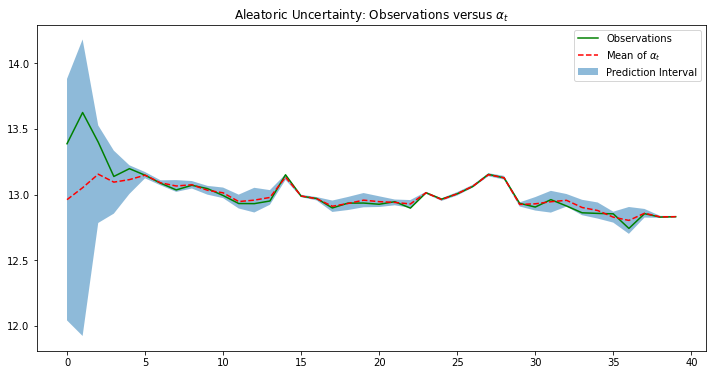As seen from the plot, the mean of $\alpha_t$ appears much smoother than before. The reason is because by fitting both the location and scale of $\text{Normal}(\alpha_t, f(\beta_t))$, we enable a more flexible fit to the data (contrast this from before when SigmaEpsilon() was a single parameter that was supposed to estimate the noise for the entire sequence!). For example, when the data becomes more noisy, our model can simply widen $\beta_t$ without overcorrecting $\alpha_t$ which ensures smoother connections with later points. Thus, the model is able to more accurately detect a latent trend while still learning an evolving (heteroskedastic) noise level. This is an example of learning the aleatoric uncertainty (inherent randomness), which we display with a prediction interval.

Now we show the other type of uncertainty, epistemic uncertainty.

epistemic_alpha = np.quantile(hetero_alpha_samples[-tail_len:].detach().numpy(), [0.05, 0.95], axis=0)# CI for Betaplt.figure(figsize=(16, 10))plt.fill_between(    torch.arange(N),     np.quantile((hetero_alpha_samples[-tail_len:] - hetero_scale_samples[-tail_len:]).detach().numpy(), 0.05, 0),    np.quantile((hetero_alpha_samples[-tail_len:] + hetero_scale_samples[-tail_len:]).detach().numpy(), 0.95, 0),     alpha=0.5,    label=r"95% Credible Interval for $\beta_t \pm \alpha_t$")# Beta samplesplt.plot(hetero_alpha_samples[-tail_len:].T - hetero_scale_samples[-tail_len:].T, 'r--', linewidth=0.1)plt.plot(hetero_alpha_samples[-tail_len:].T + hetero_scale_samples[-tail_len:].T, 'r--', linewidth=0.1)plt.plot(hetero_alpha_samples[-tail_len:].T + hetero_scale_samples[-tail_len:].T, 'r--', label=r'Samples of $\beta_t \pm \alpha_t$', linewidth=0.1)# CI for Alphaplt.fill_between(    torch.arange(N),     epistemic_alpha[0, ...],     epistemic_alpha[1, ...],     alpha=0.5,     color='red',     label=r"95% Credible Interval for $\alpha_t$")# Samples for Alphaplt.plot(hetero_alpha_samples[-tail_len:].T, 'b--', linewidth=0.3)plt.plot(hetero_alpha_samples[-tail_len:].T[0, ...], 'b--', label=r'Samples of $\alpha_t$', linewidth=0.3)plt.plot(y_obs, label="Observations", color="green", linewidth=3)plt.title(r"Epistemic Uncertainty: Observations versus $\alpha_t$")plt.legend()plt.show()